##### Actions

(diff) ← Older revision | Latest revision (diff) | Newer revision → (diff)

Graphic representations of knots and links, which basically consist of plane projections. Let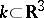be a link and letbe the projection. The order of a point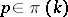is defined as the number of elements in the set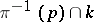. A point of order two is called a double point and a point of a order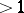a multiple point. One says that a polygonal linkis situated in regular position if: 1) all its multiple points are double and their number is finite; and 2) no double point is the image of a vertex. Every link may be transformed into regular position by an arbitrarily small rotation of space. Ifis in regular position, then for each double point the branch lying above it (in the direction of the-axis) is called an overpass and the branch lying below it an underpass. In order to give a diagram of a link in regular position, it is necessary to give its projection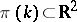and cut the image of an underpass at double points (cf. Fig. a). underpass overpass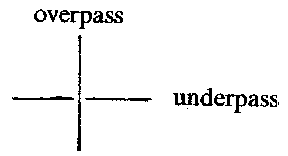Figure: k055570a

If the link is oriented, i.e. a manner for traversing each component is given, one indicates this by arrows in the diagram. If for this traversal of every component of a link the overpasses and underpasses in its projections alternate, the diagram is called alternating. A link having at least one alternating diagram is called an alternating link (cf. Alternating knots and links).

Every domain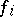into which the projection of a link divides the planehas a corresponding index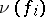, equal to the sum of the number of circuits accomplished by the projection of each component of the link about an interior point of. By transition to an adjacent domain the index changes by 1, which implies that every domainmay be coloured black or white like a chess board.

In the set of all diagrams in the plane an equivalence relation may be introduced, where two diagrams are called equivalent if and only if the links corresponding to them are isotopic (cf. Isotopy). This makes it possible to reduce the study of knots to plane topology. Thusif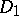may be obtained from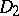by applying a finite number of the elementary operations I–III shown in Fig. band isotopic deformations.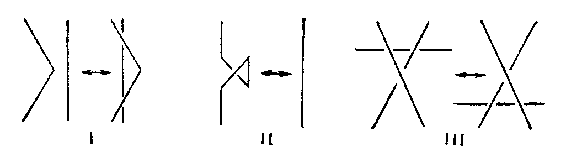Figure: k055570b

The approach to knot theory established in this way is typical of combinatorial topology. It arose in the first period of development of knot theory (roughly up to the 1940's). In the framework of this approach invariants of knots were defined starting from diagrams and it was then proved that the result did not depend on the choice of a diagram (cf. ). In the modern development the definition of invariants is preferably given in terms of algebraic topology, which strips it in first instance from the geometric essence.

The diagram of an oriented link is used in the construction of its Seifert surface. Letbe any point, not double, of an oriented diagramof a link. The diagramis traversed in the direction of the given orientation, starting at. At the first encounter of a double point a turn is effected and the motion is continued in the direction of the orientation ofuntil one returns to; thus one has described a simple closed contour, called a Seifert circle. The diagramis divided into these Seifert circles,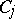, which can only touch each other. For each circleone denotes bythe disc lying in a plane parallel to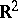such that its boundary projects onto. For each double point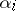one considers the rectangle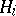placed vertically on, twisted by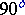so that the upper edge lies on the boundary of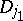and the lower on the boundary of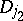, ifand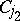are the Seifert circles meeting in. If each turn is performed to the appropriate side, then the boundary arising from the surface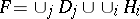is isotopic to the link. The surface so obtained is orientable. A simpler, but possibly unorientable, surface spanned by the link may be obtained by putting a disc over each black area of the chess board diagram and adding, as above, turns through right angles.

Various methods are known for describing the presentations of the group of a link by its diagram. In order to obtain the Wirtinger presentation one considers the set of connected componentsof the diagram of a link (the set of overpasses), numbered in the order of crossing each component of the link in the direction given by the orientation. Letbe a certain symbol associated with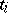. For each double point one hasor, depending on whether the diagram of the link in a neighbourhood of this double point has the form shown in Fig.3aor Fig.3b. The group given by the presentation with generators(one for each overpass) and the above-mentioned defining relations (one for each double point) is isomorphic to the group of the link considered. The presentation so obtained is called the upper Wirtinger presentation. Dually one defines the lower Wirtinger presentation.Figure: k055570c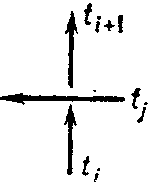Figure: k055570d

To find the Dehn presentation one considers the domainsinto which the projection of the link divides the plane and introduces a symbolfor each domain. The symbols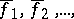are taken as generators, while the relations are obtained from traversal starting at the points: write in succession the symbols corresponding to the domains touching at, where if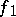and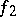lie on the left of an overpass, then the degrees of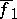andare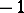whileand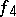equal. Moreover, put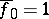, where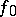is the exterior domain.

## The use of knot and link diagrams to transform links into closed braids.

(Cf. Braid theory.) Choose a pointoutsidesuch that the rays issuing from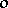contain no double points or segments from. A complete traversal of one of the componentsof the link can be effected as long as the corresponding traversal in the projection oftakes place in one and the same direction around. Letbe the first segment directed oppositely and let a pointbe taken such that the triangle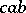, whereandare the ends of, containsin its interior and the sides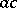andare in general position relative to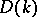. It can be shown that there is at most one double point on. Replaceinby, where ifcontains no double point or is an overpass, it is supposed thatandare overpasses at all points of their intersection with. Ifhas an underpass, one takes them to be underpasses. Extend this construction for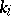and apply it in succession to all components; then a new diagram foris obtained in which the projection of each component goes aroundall the time on the same side, i.e.is represented in the form of a closed braid .

How to Cite This Entry: